JEE  >  JEE Advanced (Single Correct MCQs): Equilibrium

# JEE Advanced (Single Correct MCQs): Equilibrium - JEE

Test Description

## 42 Questions MCQ Test Chemistry for JEE Main & Advanced - JEE Advanced (Single Correct MCQs): Equilibrium

JEE Advanced (Single Correct MCQs): Equilibrium for JEE 2023 is part of Chemistry for JEE Main & Advanced preparation. The JEE Advanced (Single Correct MCQs): Equilibrium questions and answers have been prepared according to the JEE exam syllabus.The JEE Advanced (Single Correct MCQs): Equilibrium MCQs are made for JEE 2023 Exam. Find important definitions, questions, notes, meanings, examples, exercises, MCQs and online tests for JEE Advanced (Single Correct MCQs): Equilibrium below.
 1 Crore+ students have signed up on EduRev. Have you?
JEE Advanced (Single Correct MCQs): Equilibrium - Question 1

### Molten sodium chloride conducts electricitry due to the presence of

Detailed Solution for JEE Advanced (Single Correct MCQs): Equilibrium - Question 1

In molten state the cations and anions become free and flow of current is due to migration of these ions in opposite directions in the electric field.

JEE Advanced (Single Correct MCQs): Equilibrium - Question 2

### An acidic buffer solution can be prepared by mixing the solutions of

Detailed Solution for JEE Advanced (Single Correct MCQs): Equilibrium - Question 2

NOTE : Acidic buffer is mixture of weak acid and its salt with common anion.

(a) CH3COOH + CH3COONH4 is acidic buffer.
(b) NH4Cl + NH4OH is basic buffer.
(c) H2SO4 + Na2SO4 is not buffer because both the compounds are strong electrolytes.
(d) NaCl + NaOH is not buffer solution because both compounds are strong electrolytes.

JEE Advanced (Single Correct MCQs): Equilibrium - Question 3

### The pH of a 10–8 molar solution of HCl in water is

Detailed Solution for JEE Advanced (Single Correct MCQs): Equilibrium - Question 3

TIPS/Formulae :
(i) pH of acid cannot be more than 7.
(ii) While cal culating pH in such case, consider contribution [H+]  from water also.
Molar conc. of HCl = 10–8. (given)
∴ pH = 8. But this cannot be possible as pH of an acidic solution can not be more than 7. So we have to consider [H+] coming from H2O.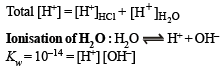Let x be the conc. of [H+] from H2O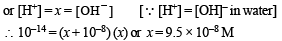[For quadratic equation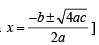∴  Total [H+] = 10–8 + 9.5 × 10–8
= 10.5 × 10–8 or  pH
= – log (10.5 × 10–8) = 6.98
It is between 6 and 7.

JEE Advanced (Single Correct MCQs): Equilibrium - Question 4

The oxidation of SO2 by O2 to SO3 is an exothermic reaction. The yield of SO3 will be maximum if

Detailed Solution for JEE Advanced (Single Correct MCQs): Equilibrium - Question 4

TIPS/Formulae :
(i) According is Le-Chateliers principle, exothermic, reactions are favoured at low temperature.
(ii) According to Le-Chateliers principle, the reaction in which n < 0, are favoured at high pressure.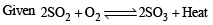∵ It is exothermic reaction
∴Yield of SO3 is maximum at low temperature
n = 2 – 3= – 1 or n < 0
∴ Yield of SO3 is maximum at high pressure.

JEE Advanced (Single Correct MCQs): Equilibrium - Question 5

For the reaction :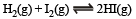the equilibrium constant Kp changes with

Detailed Solution for JEE Advanced (Single Correct MCQs): Equilibrium - Question 5

Only temperature affects the equilibrium constant. Since here ΔH = 2 – 2 = 0 , so there is no change in Kp when total pressure changes.

JEE Advanced (Single Correct MCQs): Equilibrium - Question 6

Of the given anions, the strongest Bronsted base is

Detailed Solution for JEE Advanced (Single Correct MCQs): Equilibrium - Question 6

TIPS/Formulae :

(i) Lower the oxidation state of central atom, weaker will be oxy acid.
(ii) Weaker the acid, stronger will be its conjugate base.
Oxidation state of Cl in HClO is + 1, in HClO2 is + 3, in HClO3 is + 5, and in HClO4 is + 7
∴ HClO is the weakest acid and so its conjugate base ClO is the strongest Bronsted base.

JEE Advanced (Single Correct MCQs): Equilibrium - Question 7

At 90ºC, pure water has [H3O+] 10–6 mole litre–1. What is the value of Kw at 90ºC?

Detailed Solution for JEE Advanced (Single Correct MCQs): Equilibrium - Question 7

For pure water,  [H3O+] = [OH]
⇒ Kw = 10–6 × 10–6 = 10–12

JEE Advanced (Single Correct MCQs): Equilibrium - Question 8

The precipitate of

CaF2(Ksp = 1.7 × 10–10)

is obtained when equal volumes of the following are mixed

Detailed Solution for JEE Advanced (Single Correct MCQs): Equilibrium - Question 8

TIPS/Formulae :
For precipitation to occur ionic product > solubility products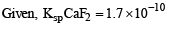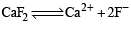Ionic product of C aF2 = [Ca2+] [F-]2 Calculate I.P. in each case

(a) I.P. of CaF2 = (10–4) × (10–4)2  = 10–12
(b) I.P. of CaF2 = (10–2) × (10–3)2  = 10–8
(c) I.P. of CaF2  =  (10–5) × (10–3)2  = 10–11
(d) I.P. of CaF2 =  (10–3) × (10–5)2  = 10–13

∵ I.P > solubility in choice (b) only..
∴ ppt of CaF2 is obtained in case of choice (b) only..

JEE Advanced (Single Correct MCQs): Equilibrium - Question 9

A liquid is in equilibrium with its vapour at its boiling point. On the average, the molecules in the two phases have equal :

Detailed Solution for JEE Advanced (Single Correct MCQs): Equilibrium - Question 9

Vapours and liquid are at the same temperature.

JEE Advanced (Single Correct MCQs): Equilibrium - Question 10

Pure ammonia is placed in a vessel at a temperature where its dissociation constant (a) is appreciable. At equilibrium :

Detailed Solution for JEE Advanced (Single Correct MCQs): Equilibrium - Question 10

Statement (a) is correct and the rest statements are wrong. Kp depends only on temperature hence at constant temp. Kp will not change.

JEE Advanced (Single Correct MCQs): Equilibrium - Question 11

A certain buffer solution contains equal concentration of X and HX. The Kb for X is 10–10. The pH of the buffer is :

Detailed Solution for JEE Advanced (Single Correct MCQs): Equilibrium - Question 11

For a basic buffer,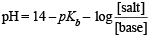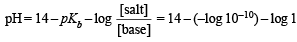⇒ pH = 4

JEE Advanced (Single Correct MCQs): Equilibrium - Question 12

A certain weak acid has a dissociation constant of 1.0 × 10–4. The equilibrium constant for its reaction with a strong base is :

Detailed Solution for JEE Advanced (Single Correct MCQs): Equilibrium - Question 12

TIPS/Formulae :

The equilibrium constant for the nuetralization of a weak acid with a strong base is given by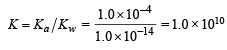JEE Advanced (Single Correct MCQs): Equilibrium - Question 13

An example of a reversible reaction is :

Detailed Solution for JEE Advanced (Single Correct MCQs): Equilibrium - Question 13

As all the reactants and products are present in aqueous form in (d) so  it is a reversible reaction. In others either solid or gas is generated which is insoluble or volatile and hence makes the reaction unidirectional.

JEE Advanced (Single Correct MCQs): Equilibrium - Question 14

The best indicator for detection of end point in titration of a weak acid and a strong base is :

Detailed Solution for JEE Advanced (Single Correct MCQs): Equilibrium - Question 14

TIPS/Formulae :

The pH of the solution at the equivalence point will be greater than 7 due to salt hydrolysis. So an indicator giving colour in basic medium will be suitable.
Phenolphthalein is a good indicator if the base is strong because strong base immediately changes the pH at end point.

JEE Advanced (Single Correct MCQs): Equilibrium - Question 15

The conjugate acid of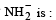Detailed Solution for JEE Advanced (Single Correct MCQs): Equilibrium - Question 15

Base + H+ → (conjugate acid) -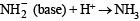(conjugate acid)

JEE Advanced (Single Correct MCQs): Equilibrium - Question 16

The compound that is not a Lewis acid is :

Detailed Solution for JEE Advanced (Single Correct MCQs): Equilibrium - Question 16

NOTE : Electron acceptors or elements having incomplete octet are Lewis acids.
(i) BF3 (B has 6 e in valance shell), AlCl3 (Al has 6 electrons in valance shell), BeCl2 (Be has 4 e in valance shell) are electron defecient compounds and hence Lewis acids.
(ii) SnCl4 has complete octet so it is Lewis base.

JEE Advanced (Single Correct MCQs): Equilibrium - Question 17

The compound insoluble in acetic acid is :

Detailed Solution for JEE Advanced (Single Correct MCQs): Equilibrium - Question 17

CaO, CaCO3 and Ca(OH)2 dissolve in CH3COOH due to formation of  (CH3COO)2Ca. But CaC2O4 does not dissolve as CH3COO is a stronger conjugate base than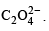JEE Advanced (Single Correct MCQs): Equilibrium - Question 18

The compound whose 0.1 M solution is basic is :

Detailed Solution for JEE Advanced (Single Correct MCQs): Equilibrium - Question 18

(a) is a neutral solution due to both cationic and anionic hydrolysis (Ka = Kb = 1.8×10–5); (b) is acidic solution due to cationic hydrolysis; (c) is acidic solution due to cationic hydrolysis; (d) is basic solution due to anionic hydrolysis.
Alternate solution.

JEE Advanced (Single Correct MCQs): Equilibrium - Question 19

When equal volumes of the following solutions are mixed, precipitation of AgCl (Ksp = 1.8×10–10) will occur only with

Detailed Solution for JEE Advanced (Single Correct MCQs): Equilibrium - Question 19

For a precipitation to occur Solubility product < Ionic product
Given  Ksp = 1.8 × 10–10
Calculating ionic products in each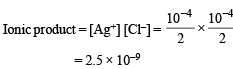which is greater than Ksp (1.8 × 10–10).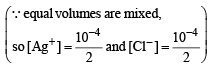JEE Advanced (Single Correct MCQs): Equilibrium - Question 20

The pKa of acetylsalicyclic and (aspirin) is 3.5. The pH of gastric juice in human stomach is about 2-3 and the pH in the small intestine is about 8. Aspirin will be

Detailed Solution for JEE Advanced (Single Correct MCQs): Equilibrium - Question 20

TIPS/Formulae :
In acidic medium weak acids are unionized due to common ion effect and they are completely ionised in alkaline medium.
Aspirin (or acetyl salicylic acid) is unionised in stomach (where pH is 2-3 ) and is completely ionised in small intestine (when pH is 8)

JEE Advanced (Single Correct MCQs): Equilibrium - Question 21

Which one of the following is the strongest acid?

Detailed Solution for JEE Advanced (Single Correct MCQs): Equilibrium - Question 21

TIPS/Formulae :
(i) Higher the electronegatively of central atom higher will be the acidic strength.
(ii) In case of same atom higher the value of oxidation state of the metal, higher will be its acidic strength.
The  electronegativity of Cl > S.
Oxidation no. of Cl in ClO3(OH) = + 7 Oxidation no. of  Cl in ClO2(OH) = + 5 Oxidation no. of S in SO(OH)2 = + 4 Oxidation no. of  S in SO2(OH)2 = + 6

∴ ClO3(OH) is the strongest acid.

JEE Advanced (Single Correct MCQs): Equilibrium - Question 22

Amongst the following hydroxides, the one which has the lowest value of Ksp at ordinary temperature (about 25ºC) is

Detailed Solution for JEE Advanced (Single Correct MCQs): Equilibrium - Question 22

NOTE : In case of alkaline earth hydroxides solubility increases on moving down the group.
Be(OH)2 has lowest solubility and hence lowest solubility product. [Be at tip of the group]

JEE Advanced (Single Correct MCQs): Equilibrium - Question 23

The reaction which proceeds in the forward direction is

Detailed Solution for JEE Advanced (Single Correct MCQs): Equilibrium - Question 23

Due to absence of hydrolysation of FeCl3 backward reaction will not take place.

JEE Advanced (Single Correct MCQs): Equilibrium - Question 24

The following equilibrium in established when hydrogen chloride is dissolved in acetic acid.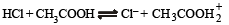The set that characterises the conjugate acid-base pairs is

Detailed Solution for JEE Advanced (Single Correct MCQs): Equilibrium - Question 24

Since HCl is stronger than CH3COOH hence acts as acid. On the other hand Cl is a stronger base than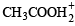and is the conjugate base of HCl.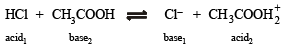JEE Advanced (Single Correct MCQs): Equilibrium - Question 25

Which of the following solutions will have pH close to 1.0?

Detailed Solution for JEE Advanced (Single Correct MCQs): Equilibrium - Question 25

(a) It is not correct answer because 100 ml M/10 HCl will completely neutralise 100 ml M/10 NaOH and the solution will be neutral.
(b) After neutralisation resultant solution will be acidic due to presence of excess of HCl.
(c) After netralisation resultant solution will be basic due to presence of excess of NaOH.
(d) M. eq. of HCl = 75 N/5 = 15 Meq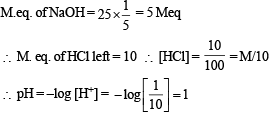JEE Advanced (Single Correct MCQs): Equilibrium - Question 26

The degree of dissociation of water at 25ºC is 1.9 × 10–7% and density is 1.0 g cm–3. The ionic constant for water is :

Detailed Solution for JEE Advanced (Single Correct MCQs): Equilibrium - Question 26

TIPS/Formulae :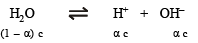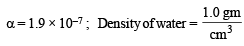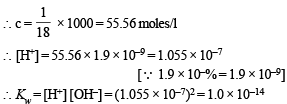JEE Advanced (Single Correct MCQs): Equilibrium - Question 27

Which one is more acidic in aqueous solution

Detailed Solution for JEE Advanced (Single Correct MCQs): Equilibrium - Question 27

Salts of weak base and strong acid get hydrolysed in aqueous solution forming an acidic solution.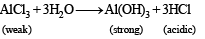JEE Advanced (Single Correct MCQs): Equilibrium - Question 28

The following acids have been arranged in the order of decreasing acid strength. Identify the correct order.

ClOH (I),   BrOH(II),  IOH(III)

Detailed Solution for JEE Advanced (Single Correct MCQs): Equilibrium - Question 28

Among  oxyacids of the same type formed by different elements, acidic nature increases with increasing electronegativity. In general, the strength of oxyacids decreases as we go down the family in the periodic table.

HOCl  (I)     >    HOBr  (II)    >    HOI (III)
[In halogen groups Cl is above Br and I]

JEE Advanced (Single Correct MCQs): Equilibrium - Question 29

The pH of 0.1 M solution of the following salts increases in the order.

Detailed Solution for JEE Advanced (Single Correct MCQs): Equilibrium - Question 29

The characteristics of the given solutions are:
NaCl – neutral solution
NH4Cl – slightly acidic due to the following reaction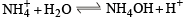NaCN – slightly alkaline due to the following reaction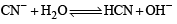HCl – highly acidic
The pH of the solution will follow the order highly acidic < slightly acidic < neutral < slightly alkaline i.e.
HCl < NH4Cl < NaCl < NaCN

JEE Advanced (Single Correct MCQs): Equilibrium - Question 30

For the chemical reaction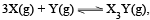the amount of X3Y at equilibrium is affected by

Detailed Solution for JEE Advanced (Single Correct MCQs): Equilibrium - Question 30

The given reaction will be exothermic in nature due to the formation of three  X - Y bonds from the gaseous atoms. The reaction is also accompanied with the decrease in the gaseous species (i.e. Dn is negative).
Hence, the reaction will be affected by both temperature and pressure. The use of catalyst does not affect the equilibrium concentrations of the species in the chemical reaction.

JEE Advanced (Single Correct MCQs): Equilibrium - Question 31

For the reversible reaction,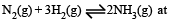500°C, the value of Kp is 1.44 x10-5 when partial pressure is measured in atmospheres. The corresponding value of Kc, with concentration in mole litre–1, is

Detailed Solution for JEE Advanced (Single Correct MCQs): Equilibrium - Question 31

Kb = Kc .( RT)Δn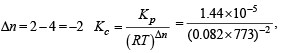(R in L.atm.K–1 mole–1).

JEE Advanced (Single Correct MCQs): Equilibrium - Question 32

When two reactants, A & B are mixed to give products C & D, the reaction quotient Q, at the initial stages of the reaction

Detailed Solution for JEE Advanced (Single Correct MCQs): Equilibrium - Question 32

At initial stage of reaction, concentration of each product will increase and hence Q will increase.

JEE Advanced (Single Correct MCQs): Equilibrium - Question 33

For a sparingly soluble salt ApBq, the relationship of its solubility product (LS) with its solubility (S) is

Detailed Solution for JEE Advanced (Single Correct MCQs): Equilibrium - Question 33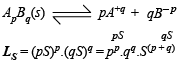JEE Advanced (Single Correct MCQs): Equilibrium - Question 34

The set with correct order of acidity is

Detailed Solution for JEE Advanced (Single Correct MCQs): Equilibrium - Question 34

TIPS/Formulae : For oxyacids containing similar central atom, the acid strength increases with the increase in the number of oxygen atom attached to the central atom and not attached to any other atom.
TIPS/Formulae : Higher the oxidation number of the central atom, higher is the acidity of the species. Thus acidity  follows the order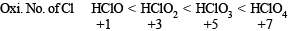JEE Advanced (Single Correct MCQs): Equilibrium - Question 35

At constant temperature, the equilibrium constant (Kp) for the decomposition reaction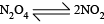is expressed by Kp = (4x2P)/(1-x2), where P = pressure, x = extent of decomposition. Which one of the following statements is true?

Detailed Solution for JEE Advanced (Single Correct MCQs): Equilibrium - Question 35

At constant temperature Kp pressure constant.
With change of pressure, x will change in such a way that Kp remains a constant.

JEE Advanced (Single Correct MCQs): Equilibrium - Question 36

Consider the following equilibrium in a closed container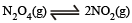At a fixed temperature, the volume of the reaction container is halved. For this change, which of the following statements holds true regarding the equilibrium constant (Kp) and degree of dissociation (α)?

Detailed Solution for JEE Advanced (Single Correct MCQs): Equilibrium - Question 36

TIPS/Formulae :

At constant temperature Kp or Kc remains constant.
For the equilibria :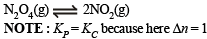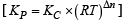Since temperature is constant so Kc or Kp will remain constant. Further since volume is halved, the pressure will be doubled so a will decrease so as to maintain the constancy of Kc or Kp.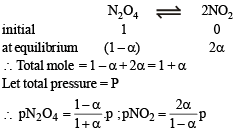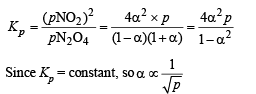So when volume is halved, pressure gets doubled and thus α will decrease.

JEE Advanced (Single Correct MCQs): Equilibrium - Question 37

A weak acid HX has the dissociation constant 1 × 10–5 M. It forms a salt NaX on reaction with alkali. The percentage hydrolysis of 0.1 M solution of NaX is

Detailed Solution for JEE Advanced (Single Correct MCQs): Equilibrium - Question 37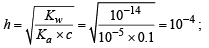Hence, % hydrolysis = 10–4 × 100 = 0.01

JEE Advanced (Single Correct MCQs): Equilibrium - Question 38

A 0.004 M solution of Na2SO4 is isotonic with 0.010 M solution of glucose at same temperature. The  percentage dissociation of Na2SO4 is

Detailed Solution for JEE Advanced (Single Correct MCQs): Equilibrium - Question 38

NOTE : For isotonic solutions, osmotic pressure is same.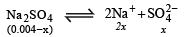Since both solutions are isotonic, therefore, 0.004 + 2x = 0.01 ; x = 3 × 10–3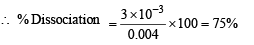Alternate eqn. Total no. of moles = 0.004 × x + 2x + x

JEE Advanced (Single Correct MCQs): Equilibrium - Question 39

0.1 mole of CH3NH2 (Kb = 5 x10–4)is mixed with 0.08 mole of HCl and diluted to one litre. What will be the H+ concentration in the solution?

Detailed Solution for JEE Advanced (Single Correct MCQs): Equilibrium - Question 39

NOTE : For basic buffer pH is more than 7.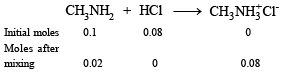As it is a basic buffer solution.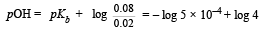=  3.30  +  0.602  = 3.902
pH  = 14 – 3.902 = 10.09; [H+]  = 7.99 × 10–11 ≈ 8 ×10–11 M

JEE Advanced (Single Correct MCQs): Equilibrium - Question 40

The Haber ’s process for the formation of NH3 at 298K is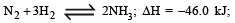Wh ich of the following is the correct statement

Detailed Solution for JEE Advanced (Single Correct MCQs): Equilibrium - Question 40

In a reversible reaction, catalyst speeds up both the forward and backward reactions to the same extent, so (c) is wrong. At equilibrium,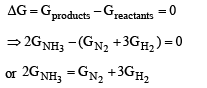JEE Advanced (Single Correct MCQs): Equilibrium - Question 41

2.5 ml of (2/5) M weak monoacidic base (Kb = 1 × 10–12 at 25°) is titrated (2/15) M HCl in water at 25°C. The concentration  of H+ at equivalence point is (Kw = 1 × 10–14 at 25°C)

Detailed Solution for JEE Advanced (Single Correct MCQs): Equilibrium - Question 41

Let the weak manoacidic base be BOH, then the reaction that occurs during titration is

BOH + HCl → BCl + H2O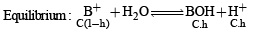Using the normality equation,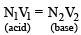Substituting various given values, we get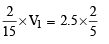Then the concentration of BCl in resulting solution is given by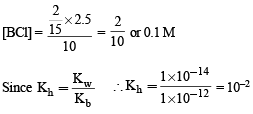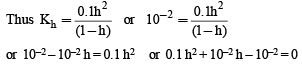(Solving this quadratic equation for h, we get)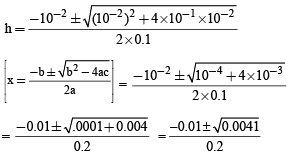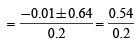[Neglecting the negative term]

= 0.27
∴ [H+] = C. h  = 0.1 × 0.27  = 2.7 × 10–2 M

Thus the correct answer is [d].

JEE Advanced (Single Correct MCQs): Equilibrium - Question 42

Solubility product constant (Ksp) of salts of types MX, MX2 and M3X at temperature T are 4.0 × 10–8, 3.2 × 10–14 & 2.7 × 10–15, respectively. Solubilities (mol dm–3) of the salts at temperature 'T' are in the order –

Detailed Solution for JEE Advanced (Single Correct MCQs): Equilibrium - Question 42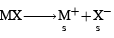(Where s is the solubility)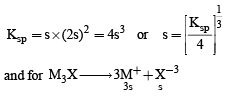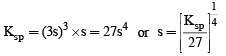From the given values of Ksp for MX, MX2 and M3X, we can find the solubilities of those salts at temperature, T.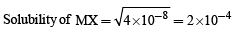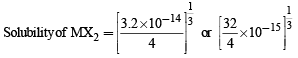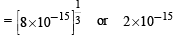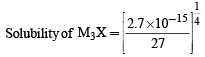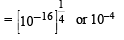Thus the solubilities are in the order MX > M3 X> MX2 i.e the correct anser is (d).

## Chemistry for JEE Main & Advanced

320 videos|585 docs|368 tests(Scan QR code)